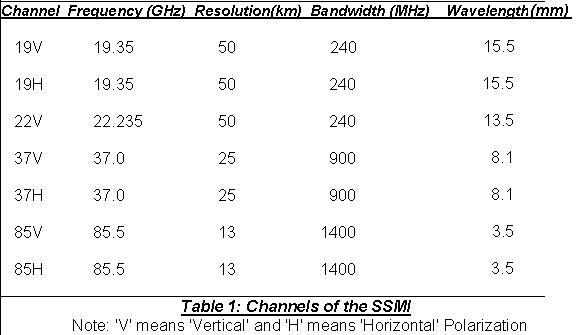## 1. Sensor Channels and Polarizations

The SSMI has four frequencies: 19.35 GigaHertz (GHz), 22.235 GHz, 37.0 GHz, and 85.5 GHz. The sensor is a passive suite: it only measures the radiation coming up from the earth. It does not send out a signal , such as a RADAR. The 19, 37, and 85 GHz frequencies are split into two channels each : one channel detects vertically polarized microwave energy, while the other receives horizontally polarized energy. The 22 GHz frequency only has a vertically polarized channel. Thus, the SSMI has seven total channels, summarized below in Table 1.The sensitivity of these channels ranges from +/- 0.37 degrees K to 0.73 K. The SSMI channels measure a quantity known as brightness temperature . Brightness temperature, or Tb, is the radiometric temperature of the earth. It is directly related to the actual temperature, via equation (1):

#### Tb = e T (1)

where T is the Temperature, and -e- is the emissivity of the earth's surface. Emissivity is a relative, dimensionless measure than varies from 0 to 1. Objects with a low emissivity (such as water ) will appear colder to the SSMI than they actually are. Conversely, objects with a high emissivity will appear closer to the actual temperature. In the ideal case, an object with a emissivity of 1 (referred to as a Planck blackbody) would emit a brightness temperature which exactly equalled the surface temperature. This is a perfect emitter.
With this in mind , one can say identify two primary functions of the SSMI : it conducts radiometry (the measure of radiant energy) and polarimetry (the measure of polarization properties of a scene) of the earth's surface.

Go to the next section.